# {ACCOUNT TOTAL:x} Supports sub { } but {ACCOUNT BALANCE:x} Does Not

Original post was another question but can delete first post, changed title as solution to original question raised different issue.

Account total if obviously sales/order/transfer total, there is obviously account balance but is not show on report tag help!
@emre not sure if you want to add that one to report tag list…

ALSO
Whats the difference then between ACCOUNT TOTAL and ACCOUNT DEBIT TOTAL?

@emre also {ACCOUNT TOTAL:x} supports sub { };

``````{ACCOUNT TOTAL:{REPORT ENTITY DETAIL:EC.Booking Number:(EN=Room 1)}}
``````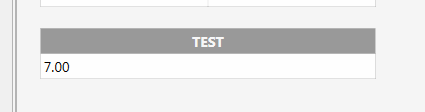{ACCOUNT BALANCE:x} doesn’t ;

``````{ACCOUNT BALANCE:{REPORT ENTITY DETAIL:EC.Booking Number:(EN=Room 1)}}
``````But expression should work as;

``````{ACCOUNT BALANCE:ZMZJ-4492672220}
``````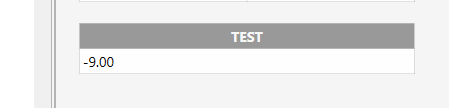These are all based on;

``````{REPORT ENTITY DETAIL:EC.Booking Number:(EN=Room 1)}
``````

which returnsALSO;
Whats up with this? {ACCOUNT CREDIT TOTAL:x}…

``````{ACCOUNT DEBIT TOTAL:{REPORT ENTITY DETAIL:EC.Booking Number:(EN=Room 1)}}
{ACCOUNT CREDIT TOTAL:{REPORT ENTITY DETAIL:EC.Booking Number:(EN=Room 1)}}
{ACCOUNT DEBIT TOTAL:ZMZJ-4492672220}
{ACCOUNT CREDIT TOTAL:ZMZJ-4492672220}
``````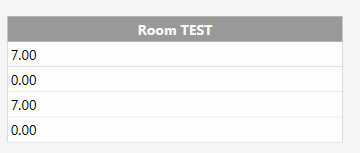I’m sure there is a way to get the balance using Report Tags, but I wouldn’t know what that is.

SQL to the rescue…

``````SELECT
e.[Name] as [EntityNname]
,a.[Name] as [AccountName]

,[Date]
,[Debit]
,[Credit]
,[Exchange]

FROM [dbo].[AccountTransactionValues] tv
LEFT JOIN [Accounts] a ON a.[Id] = tv.[AccountId]
LEFT JOIN [Entities] e ON e.[AccountId] = a.[Id]

WHERE 1=1
--AND e.[Name] = 'Celeste' -- use the Entity Name here
AND a.[Name] = '1111 1111-Celeste' -- or use the Account Name here
-- AND [Date] >= '' AND [Date] <= '' -- constrain the Date of the report
``````

So let’s make some quick mods to the query to get the Balance. Couple ways to do this:

Wrap the original inside an outer Aggregate query:

``````SELECT
-- use 1 of the following to get the balance to return a single result
-- you won't want to use both in a script unless you know how to parse
-- out multiple columns in the return
sum([Debit])-sum([Credit]) as [Balance] -- this should give the same value as below
, sum([Exchange]) as [BalanceExch] -- this should give the same value as above
FROM (

SELECT
e.[Name] as [EntityNname]
,a.[Name] as [AccountName]

,[Date]
,[Debit]
,[Credit]
,[Exchange]

FROM [dbo].[AccountTransactionValues] tv
LEFT JOIN [Accounts] a ON a.[Id] = tv.[AccountId]
LEFT JOIN [Entities] e ON e.[AccountId] = a.[Id]

WHERE 1=1
--AND e.[Name] = 'Celeste' -- use the Entity Name here
AND a.[Name] = '1111 1111-Celeste' -- or use the Account Name here
-- AND [Date] >= '' AND [Date] <= '' -- constrain the Date of the report

) balance
``````

Or modify the original to pull only values we want, and do the aggregate on the first pass:

``````SELECT
-- use 1 of the following to get the balance to return a single result
-- you won't want to use both in a script unless you know how to parse
-- out multiple columns in the return
sum([Debit])-sum([Credit]) as [Balance]
, sum([Exchange]) as [BalanceExch]

FROM [dbo].[AccountTransactionValues] tv
LEFT JOIN [Accounts] a ON a.[Id] = tv.[AccountId]
LEFT JOIN [Entities] e ON e.[AccountId] = a.[Id]

WHERE 1=1
--AND e.[Name] = 'Celeste' -- use the Entity Name here
AND a.[Name] = '1111 1111-Celeste' -- or use the Account Name here
-- AND [Date] >= '' AND [Date] <= '' -- costrain the Date of the report
``````
1 Like

Those tags are probably only using most recent Workperiod. To get the balance, you would subtract Debit-Credit. But I am betting missing \$2 is from another WP.

Look up a the SQL. It allows you to use a date range.

There is but there seems to be a bug/glitch as sure im getting the tags right…is on my list of things to learn more on.

Only got the oneCan i be super cheaky as will take you seconds and am in a real pintch on time for this one which is being shipped tomorrow, any chance you would show that in a script which returns the balance if I call the script using account name for value?

Either of the last 2 queries above will do that for you. This is the simplest shortest way…

``````SELECT
sum([Debit])-sum([Credit]) as [Balance]

FROM [dbo].[AccountTransactionValues] tv
LEFT JOIN [Accounts] a ON a.[Id] = tv.[AccountId]
LEFT JOIN [Entities] e ON e.[AccountId] = a.[Id]

WHERE 1=1
AND a.[Name] = '1111 1111-Celeste' -- use the Account Name here
-- AND [Date] >= '' AND [Date] <= '' -- constrain the Date of the report
``````

sorry @QMcKay my head is a mess trying to get this bloody setup sorted;

``````     function accountbalance()
{
var qry = "\
SELECT \
sum([Debit])-sum([Credit]) as [Balance] \
\
FROM [dbo].[AccountTransactionValues] tv \
LEFT JOIN [Accounts] a ON a.[Id] = tv.[AccountId] \
LEFT JOIN [Entities] e ON e.[AccountId] = a.[Id] \
\
WHERE 1=1 \
AND a.[Name] = 'ZMZJ-4492672220'";

var balance = sql.Query(qry);

return balance;

}

What have i missed.....

GOT IT.... :smile:

function accountbalance()
{
var qry = "\
SELECT \
sum([Debit])-sum([Credit]) as [Balance] \
\
FROM [dbo].[AccountTransactionValues] tv \
LEFT JOIN [Accounts] a ON a.[Id] = tv.[AccountId] \
LEFT JOIN [Entities] e ON e.[AccountId] = a.[Id] \
\
WHERE 1=1 \
AND a.[Name] = 'ZMZJ-4492672220'";

var r = sql.Query(qry).All;
var balance = r;
return balance;

}
``````

the r was off the bottom of the screen…

Add `.First` to the query helper.

``````var balance = sql.Query(qry).First;
``````
1 Like

Thanks, thats better than the r

Am getting the SQL code slowlyThen just make it dynamic, to use in {CALL:X}

``````function accountbalance(accountName) {
var qry = "";
qry += "SELECT ( sum([Debit]) - sum([Credit]) ) as [Balance]";
qry += " FROM [AccountTransactionValues] tv";
qry += " LEFT JOIN [Accounts] a ON a.[Id] = tv.[AccountId]";
qry += " LEFT JOIN [Entities] e ON e.[AccountId] = a.[Id]";
qry += " WHERE 1=1";
qry += " AND a.[Name] = '" + accountName + "'";

var balance = sql.Query(qry).First;

return balance;
}
``````

Cool… might use this!

``````{CALL:db.accountbalance('1111 1111-Celeste')}
``````

P.S. you don’t need the join to [Entities] if you are simply using the Account Name, so you can omit that part. I put it in there because I would rather access my Entity Balance via the Entity Name rather than the Account Name.

1 Like

Thanks @QMcKay your awesome1 Like

Can you just remind me how to do more than but not equal to in an action constraint?
Sure you told me before but for love nor money can I find the post.

Would be something like:

``````[=TN('Account Balance')] > 0
``````

or …

``[=TN('{CALL:db.accountbalance('1111 1111-Celeste')}')] > 0``
1 Like

Awesome thanks.

Does {CALL:x} not work in an ask question message?

What message? There is no message in an Automation Command.

Sorry just corrected myself

Yes, CALL:X works in ask question action …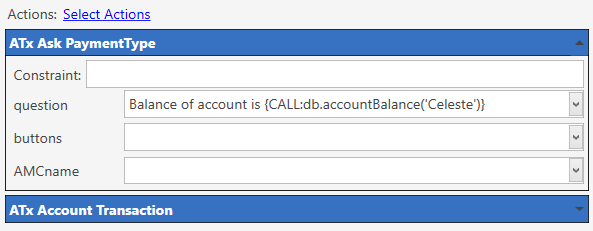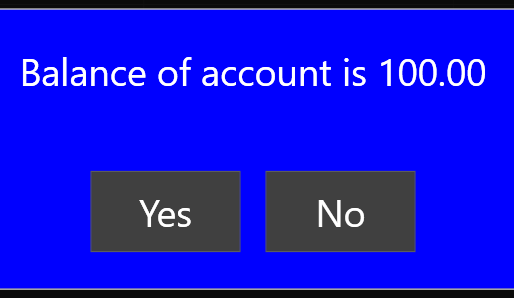Made script work with Account or Entity Name …

``````function accountBalance(entityName,accountName) {
if (typeof accountName =='undefined') {
accountName = "";
}
if (typeof entityName =='undefined') {
entityName = "";
}

var qry = "";
qry += "SELECT sum([Debit])-sum([Credit]) as [Balance]";
qry += " FROM [AccountTransactionValues] tv";
qry += " LEFT JOIN [Accounts] a ON a.[Id] = tv.[AccountId]";
qry += " LEFT JOIN [Entities] e ON e.[AccountId] = a.[Id]";
qry += " WHERE 1=1";
if (accountName != "") {
qry += " AND a.[Name] = '" + accountName + "'";
}
if (entityName != "") {
qry += " AND e.[Name] = '" + entityName + "'";
}

var balance = sql.Query(qry).First;

return balance;
}

``````

Ok, must be my use of sub { };

[=TN({CALL:accountinfo.accountbalance(’{REPORT ENTITY DETAIL:EC.Booking Number:(EN=[:CommandValue)}’)})]

This returns nothing… blank, not 0 or anything.
But works fine in the action constraint for that ask question…